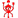Дабаби ᴿᵃⁱᵈᵉⁿㅤㅤㅤㅤㅤㅤㅤㅤㅤㅤㅤㅤㅤㅤㅤㅤ
Currently Online
Felehugyfelegeci Jul 23 @ 1:28am
-rep
Knifey McStab Jul 20 @ 5:25pm
x = 2y - 7 Formula (relating x and y)
a2 + b2 = c2 Formula (relating a, b and c)
x/2 + 7 = 0 Not a Formula (just an equation)
Example: Rearrange the volume of a box formula (V = lwh) so that the width is the subject
divide both sides by h: V/h = lw
divide both sides by l: V/(hl) = w
swap sides: w = V/(hl)
So if we want a box with a volume of 12, a length of 2, and a height of 2, we can calculate its width:

w = V/(hl)
= 12 / (2 × 2)
= 12 / 4
= 3
=Büdös Kurva Anyádat
Alika Jun 28 @ 10:29am
+rep Good AIM PRŰŰŰ
PROUVE' Jun 11 @ 5:50am
+rep parésztésönfályv❤️
levu vl. Jun 10 @ 11:24am
+rep TKzza rekeszt
Patriko Jun 8 @ 11:02pm
Helloszia. Majom himlő nincs eladó véletlen?